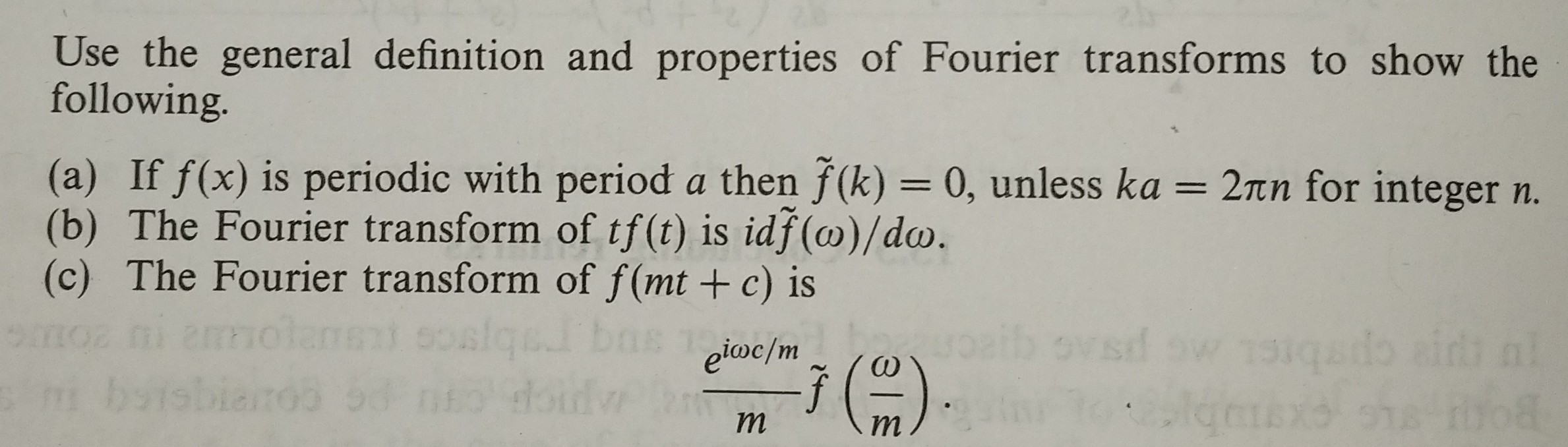# Use the general definition and properties of Fourier transforms to show thefollowing(a) If f(x) is periodic with period a then f(k)- 0, unless ka(b) The Fourier transform of tf(t) is idf(co)/do.(c) The Fourier transform of f(mt +c) is2πη for integer n.

Question
12 views

See picture below:

Please label answer clearly. Use the ~ symbol for the transformed function above the function label. For example:

~ f(w)

write the ~ symbol above the function f letter. Also label each section as a, b and c. Thank you.help_outlineImage TranscriptioncloseUse the general definition and properties of Fourier transforms to show the following (a) If f(x) is periodic with period a then f(k)- 0, unless ka (b) The Fourier transform of tf(t) is idf(co)/do. (c) The Fourier transform of f(mt +c) is 2πη for integer n. fullscreen
check_circle

Step 1

To establish the required properties of the Fourier transform of a general function f(t)

Step 2

Start with the definition of the fourier transform of a function f(t)

Step 3

(a) Assume the period is 2pi (a=2pi), for notational simplic...

### Want to see the full answer?

See Solution

#### Want to see this answer and more?

Solutions are written by subject experts who are available 24/7. Questions are typically answered within 1 hour.*

See Solution
*Response times may vary by subject and question.
Tagged in

### Math